Like   Tweet
 /* styles */ June 18, 2020 Dear Readers, As I looked back over the finalists from 2011 for the ninth installment of “Expand Your Horizons While Sheltering at Home” from the Dayton Literary Peace Prize, I was amazed by how vividly I remembered the stories, both fiction and nonfiction. This was a year of people on the move—most moved physically, some moved philosophically, but in all change led to understanding and freedom. Many of the moves were due to war: South Koreans heading south, desperate to move to safety as invaders from the north destroy their homes; a WWI veteran who moved Armenians from Turkey comes to terms with his guilt many years later; a volunteer at a Nepalese orphanage despairs that the children’s parents believe they have been moved to safety; a pilot is shot down and survives a harrowing sea voyage only to become a prisoner of war. Others move seeking freedom from social injustice including the story of an Ethiopian couple who find a new life in America. Blacks during the Great Migration work their way north and west to find relief from the oppressions of the south. In both fiction and nonfiction, the reader finds stories of transformational change: a people rebel to escape the Lion’s Gaze; a midwestern woman volunteers on the Burma/Thailand border and is changed forever; a young murderer spends decades in Angola prison and upon release is a reader, a journalist, an award-winning filmmaker; a young man returns to his homeland and comes to understand the Arab/ Israeli conflict; a financial whiz from Qatar understands the consequences of greed. Just by reviewing the titles, I became excited for readers who have yet to discover this wonderful collection of books. Every narrator in the list experiences a new awareness of self and a recognition of the humanity of others. Stay safe. Be well. Read books.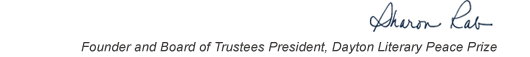table div table+table+table+table div table{width:100%;padding:0}table div table+table+table+table div table img{width:96.23%;padding:0;float:none}table div table+table+table+table div table td{width:100%;padding:0 1.88% 18px}/* styles */This list includes the winners and runners-up for 2011 and we have rich background information on each one on our website.

▪ Under Past Winners. scroll down to the year and click on it.
▪ If you click on the winners and runners-up, you will find the author’s photo and bio, a selection from the book, the judges’ citation, the writer’s reflection on literature and peace, and the option of watching the introduction and acceptance speech. If you click on the finalists, you will find a short description of each book.
 ▪ Under Past Winners. scroll down to the year and click on it.
 ▪ If you click on the winners and runners-up, you will find the author’s photo and bio, a selection from the book, the judges’ citation, the writer’s reflection on literature and peace, and the option of watching the introduction and acceptance speech. If you click on the finalists, you will find a short description of each book.

On the bottom right of the home page, you can click on COREScholar, which is built by the Chair of our Curriculum Committee Carol Loranger, Associate Dean of the College of Liberal Arts at Wright State, and maintained for us by Wright State University. There you can find interviews, TED Talks, articles, other books, films, etc. by and about each of our winning and runner-up authors. It is a great resource for students, book clubs, and readers who would like to explore an author in depth.

 table div table+table+table+table+table+table div table{width:100%;padding:0}table div table+table+table+table+table+table div table img{width:96.23%;padding:0;float:none}table div table+table+table+table+table+table div table td{width:100%;padding:0 1.88% 18px}/* styles *//* styles */ “Read global; buy local.” — Marlon James, DLPP 2009 Fiction Winner for The Book of Night Women
 table div table+table+table+table+table+table+table+table div table{width:100%;padding:0}table div table+table+table+table+table+table+table+table div table img{width:96.23%;padding:0;float:none}table div table+table+table+table+table+table+table+table div table td{width:100%;padding:0 1.88% 18px}/* styles */## 2011 Finalists - Fiction

 table div table+table+table+table+table+table+table+table+table+table div table{width:100%;padding:0}table div table+table+table+table+table+table+table+table+table+table div table img{width:96.23%;padding:0;float:none}table div table+table+table+table+table+table+table+table+table+table div table td{width:100%;padding:0 1.88% 18px}/* styles */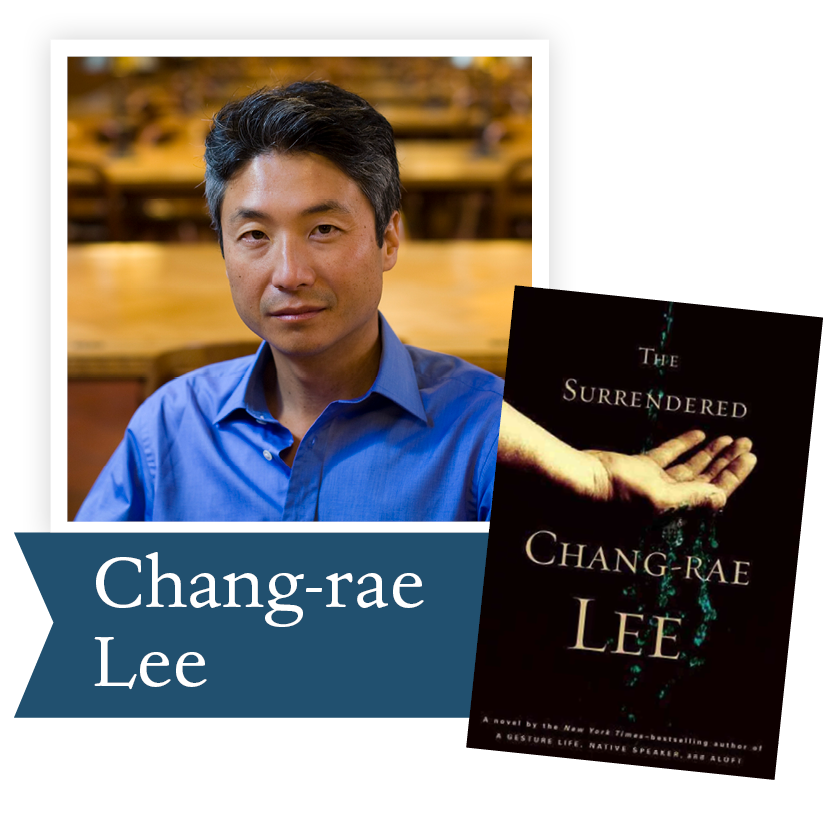The Surrendered by Chang-rae Lee (Riverhead Books): The lives of a Korean War orphan and a young GI collide in an orphanage where they vie for the attentions of a beautiful yet deeply damaged missionary wife whose elusive love seemed to transform everything.

 table div table+table+table+table+table+table+table+table+table+table+table+table div table{width:100%;padding:0}table div table+table+table+table+table+table+table+table+table+table+table+table div table img{width:96.23%;padding:0;float:none}table div table+table+table+table+table+table+table+table+table+table+table+table div table td{width:100%;padding:0 1.88% 18px}/* styles */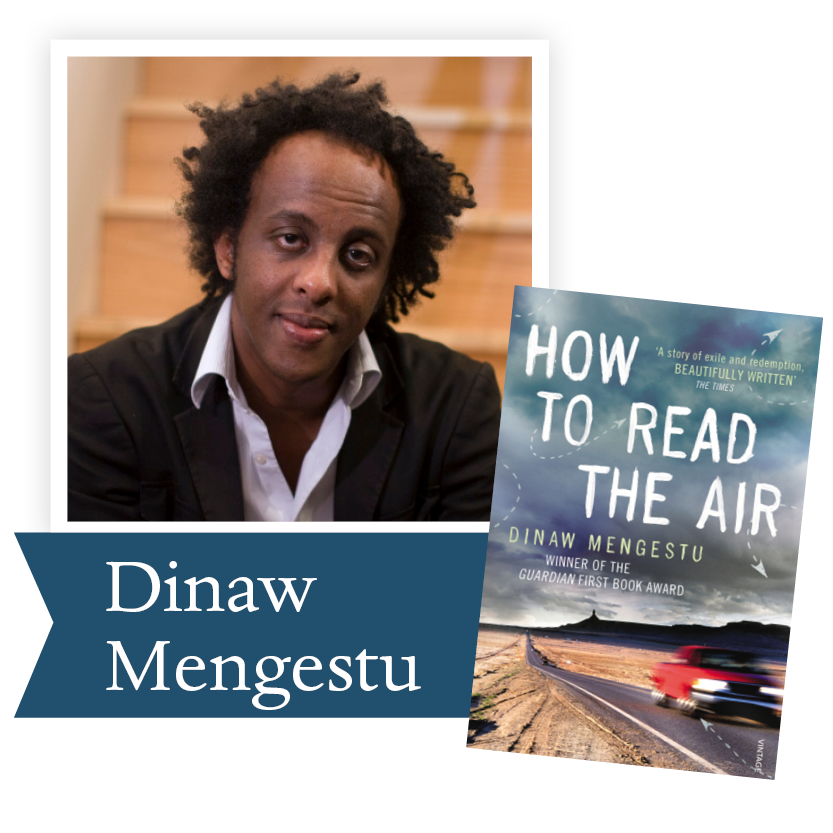How to Read the Air by Dinaw Mengestu (Riverhead Books): A young man leaves behind his marriage and job in New York to retrace his parents’ honeymoon as young Ethiopian immigrants, weaving together a family history that will take him from the war-torn country of his parents' youth to a brighter vision of his life in America today.

 table div table+table+table+table+table+table+table+table+table+table+table+table+table+table div table{width:100%;padding:0}table div table+table+table+table+table+table+table+table+table+table+table+table+table+table div table img{width:96.23%;padding:0;float:none}table div table+table+table+table+table+table+table+table+table+table+table+table+table+table div table td{width:100%;padding:0 1.88% 18px}/* styles */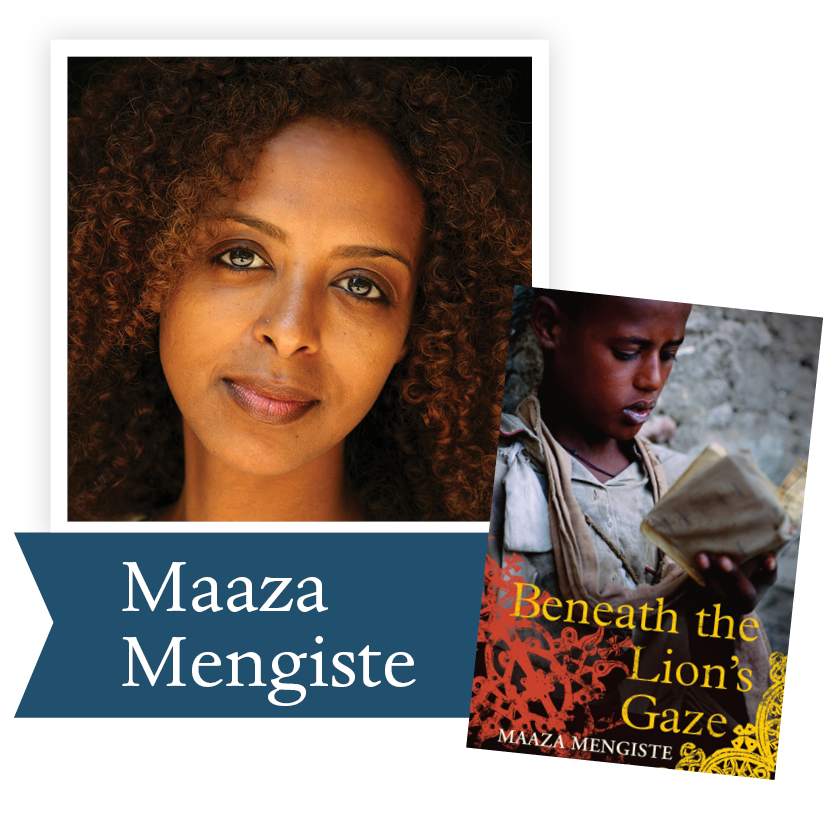Beneath the Lions Gaze by Maaza Mengiste (W. W. Norton and Company): An epic tale of a father and two sons, of betrayals and loyalties, and a family unraveling in the wake of Ethiopia’s revolution.

 table div table+table+table+table+table+table+table+table+table+table+table+table+table+table+table+table div table{width:100%;padding:0}table div table+table+table+table+table+table+table+table+table+table+table+table+table+table+table+table div table img{width:96.23%;padding:0;float:none}table div table+table+table+table+table+table+table+table+table+table+table+table+table+table+table+table div table td{width:100%;padding:0 1.88% 18px}/* styles */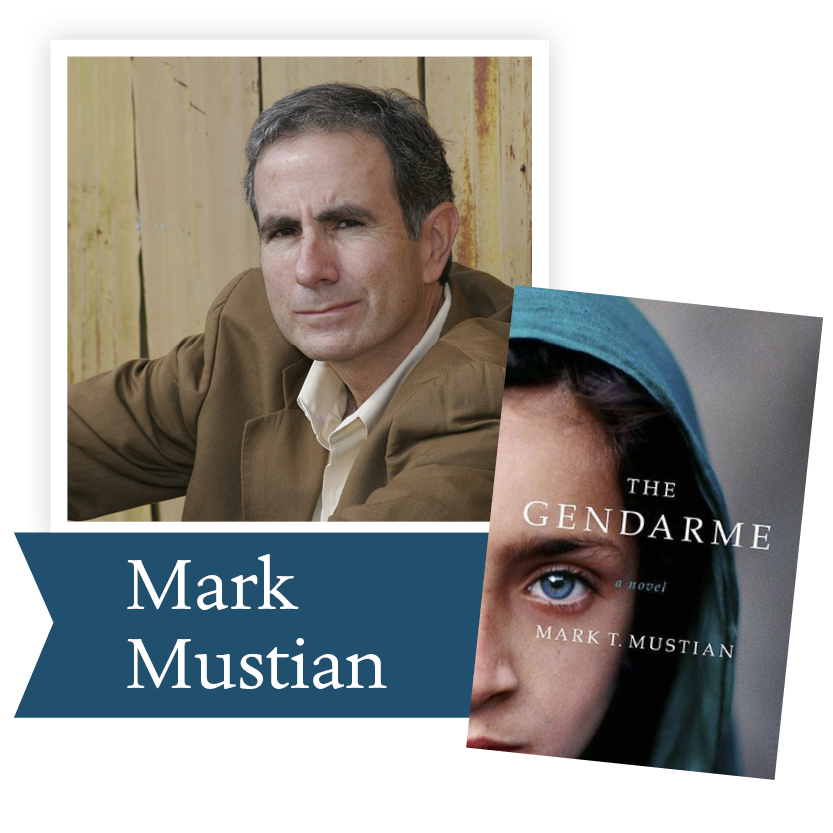The Gendarme by Mark Mustian (Amy Einhorn Books/Putnam): A World War I veteran, nearing the end of his life, is suddenly beset by memories of escorting Armenians from Turkey, churning up troubling details he and others have denied or purposely forgotten.

 table div table+table+table+table+table+table+table+table+table+table+table+table+table+table+table+table+table+table div table{width:100%;padding:0}table div table+table+table+table+table+table+table+table+table+table+table+table+table+table+table+table+table+table div table img{width:96.23%;padding:0;float:none}table div table+table+table+table+table+table+table+table+table+table+table+table+table+table+table+table+table+table div table td{width:100%;padding:0 1.88% 18px}/* styles */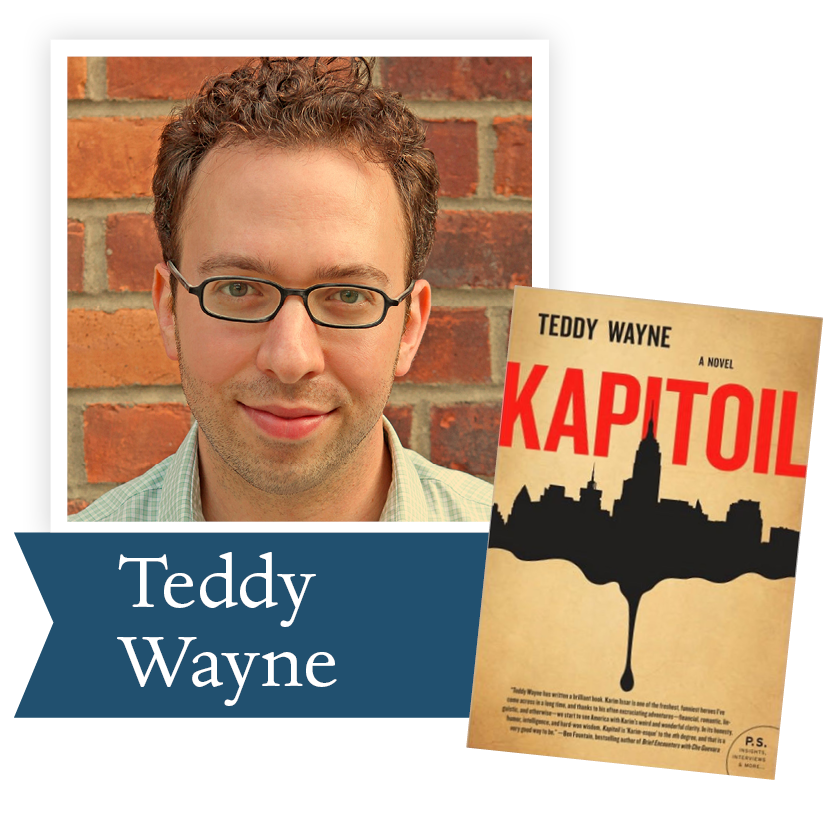Kapitoil by Teddy Wayne (HarperCollins Publishers): A young financial wizard from Qatar, fluent in numbers yet baffled by human connections, creates a computer program that predicts oil futures and reaps record profits for his American company – but carries heavy moral implications that force him to examine his loyalties.

 table div table+table+table+table+table+table+table+table+table+table+table+table+table+table+table+table+table+table+table+table div table{width:100%;padding:0}table div table+table+table+table+table+table+table+table+table+table+table+table+table+table+table+table+table+table+table+table div table img{width:96.23%;padding:0;float:none}table div table+table+table+table+table+table+table+table+table+table+table+table+table+table+table+table+table+table+table+table div table td{width:100%;padding:0 1.88% 18px}/* styles */## 2011 Finalists - Nonfiction

 table div table+table+table+table+table+table+table+table+table+table+table+table+table+table+table+table+table+table+table+table+table+table div table{width:100%;padding:0}table div table+table+table+table+table+table+table+table+table+table+table+table+table+table+table+table+table+table+table+table+table+table div table img{width:96.23%;padding:0;float:none}table div table+table+table+table+table+table+table+table+table+table+table+table+table+table+table+table+table+table+table+table+table+table div table td{width:100%;padding:0 1.88% 18px}/* styles */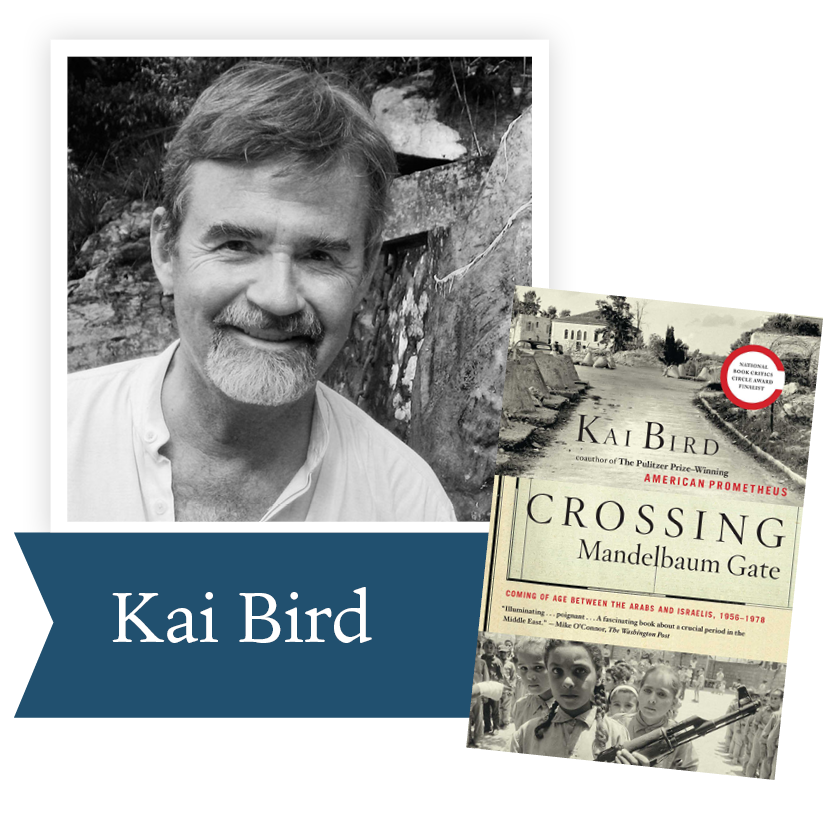Crossing Madelbaum Gate by Kai Bird (Scribner): Pulitzer Prize-winner Kai Bird’s memoir of his early years spent in Israel, Jordan, Egypt, Saudi Arabia, and Lebanon provides an original and illuminating perspective on the Arab-Israeli conflict.

 table div table+table+table+table+table+table+table+table+table+table+table+table+table+table+table+table+table+table+table+table+table+table+table+table div table{width:100%;padding:0}table div table+table+table+table+table+table+table+table+table+table+table+table+table+table+table+table+table+table+table+table+table+table+table+table div table img{width:96.23%;padding:0;float:none}table div table+table+table+table+table+table+table+table+table+table+table+table+table+table+table+table+table+table+table+table+table+table+table+table div table td{width:100%;padding:0 1.88% 18px}/* styles */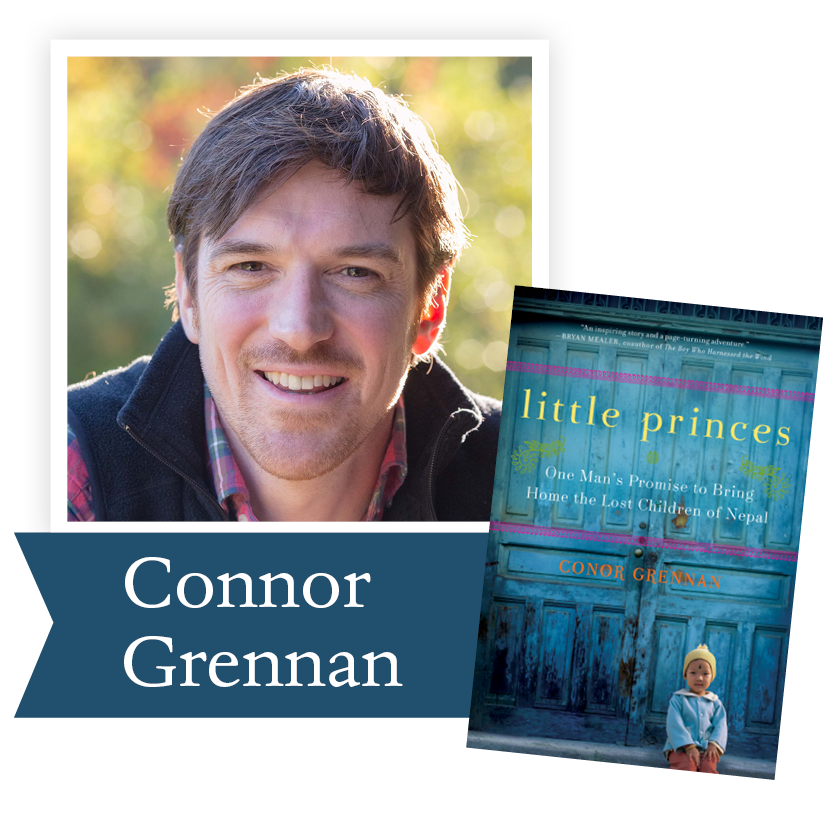Little Princes by Conor Grennan (HarperCollins Publishers): After trading his day job for a life of globe-trekking adventure, the author finds a greater purpose when he volunteers at a Nepalese “orphanage” full of children whose families believe they’ve been led out of the war-torn country to safety.

 table div table+table+table+table+table+table+table+table+table+table+table+table+table+table+table+table+table+table+table+table+table+table+table+table+table+table div table{width:100%;padding:0}table div table+table+table+table+table+table+table+table+table+table+table+table+table+table+table+table+table+table+table+table+table+table+table+table+table+table div table img{width:96.23%;padding:0;float:none}table div table+table+table+table+table+table+table+table+table+table+table+table+table+table+table+table+table+table+table+table+table+table+table+table+table+table div table td{width:100%;padding:0 1.88% 18px}/* styles */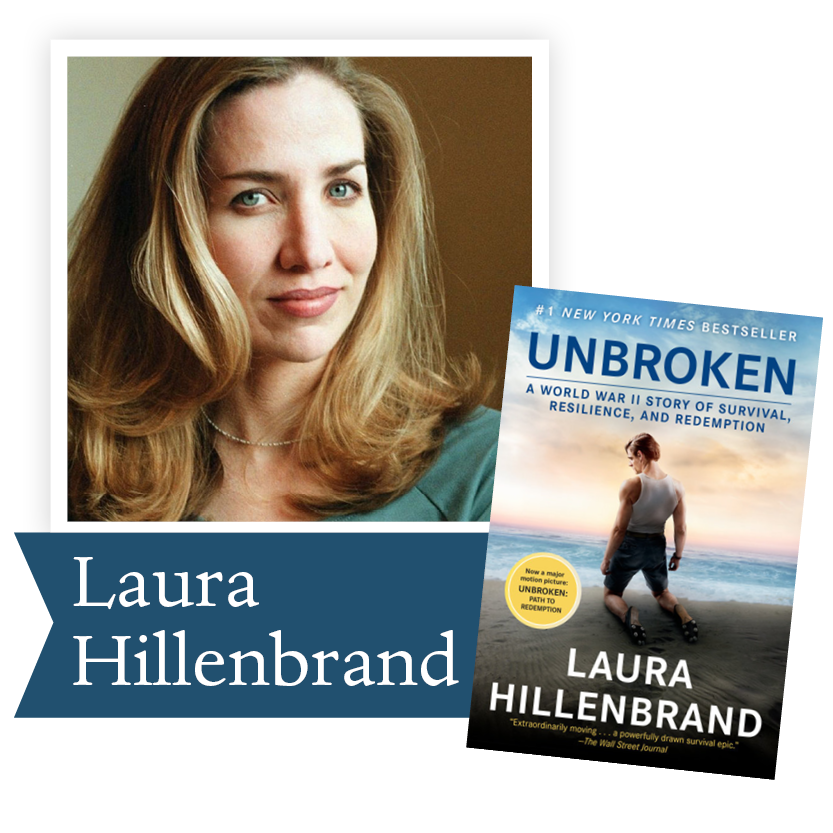Unbroken by Laura Hillenbrand (Random House): The bestselling author of Seabiscuit offers a vivid account of Louis Zamperini, a former Olympic runner who was shot down over the Pacific during World War II and drew on deep wellsprings of ingenuity, optimism, and humor to survive thousands of miles across the ocean followed by even greater trials as a prisoner of war.

 table div table+table+table+table+table+table+table+table+table+table+table+table+table+table+table+table+table+table+table+table+table+table+table+table+table+table+table+table div table{width:100%;padding:0}table div table+table+table+table+table+table+table+table+table+table+table+table+table+table+table+table+table+table+table+table+table+table+table+table+table+table+table+table div table img{width:96.23%;padding:0;float:none}table div table+table+table+table+table+table+table+table+table+table+table+table+table+table+table+table+table+table+table+table+table+table+table+table+table+table+table+table div table td{width:100%;padding:0 1.88% 18px}/* styles */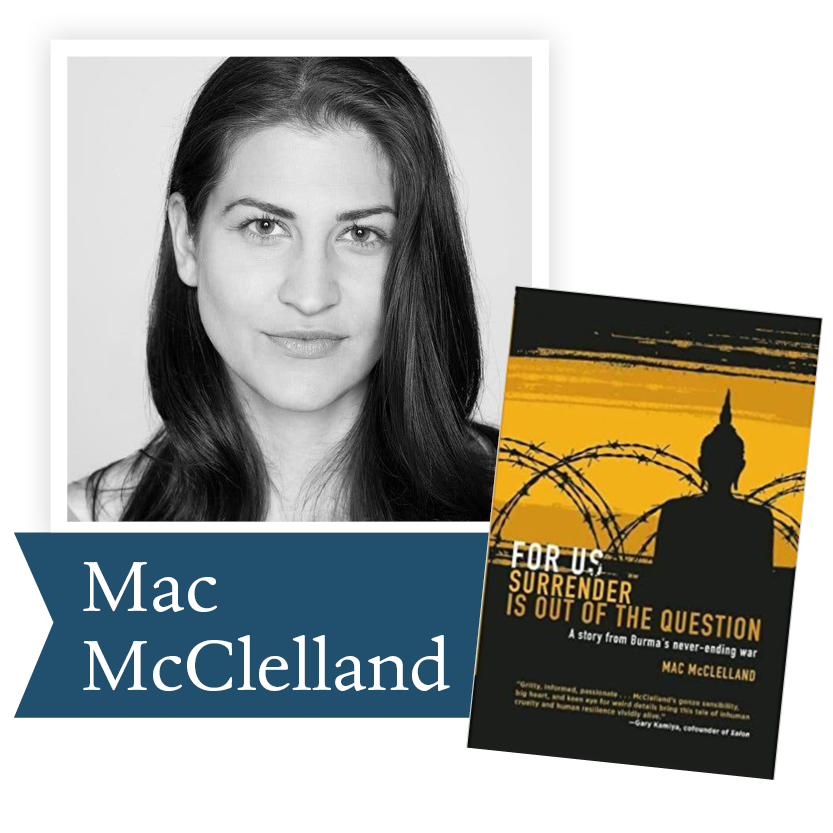For Us Surrender is Out of the Question by Mac McClelland (Soft Skull Press): Part investigative journalism, part memoir, McClelland’s fascinating debut recalls her experiences as a Midwestern twenty-something girl illegally aiding refugee activists on the Burma-Thailand border.

 table div table+table+table+table+table+table+table+table+table+table+table+table+table+table+table+table+table+table+table+table+table+table+table+table+table+table+table+table+table+table div table{width:100%;padding:0}table div table+table+table+table+table+table+table+table+table+table+table+table+table+table+table+table+table+table+table+table+table+table+table+table+table+table+table+table+table+table div table img{width:96.23%;padding:0;float:none}table div table+table+table+table+table+table+table+table+table+table+table+table+table+table+table+table+table+table+table+table+table+table+table+table+table+table+table+table+table+table div table td{width:100%;padding:0 1.88% 18px}/* styles */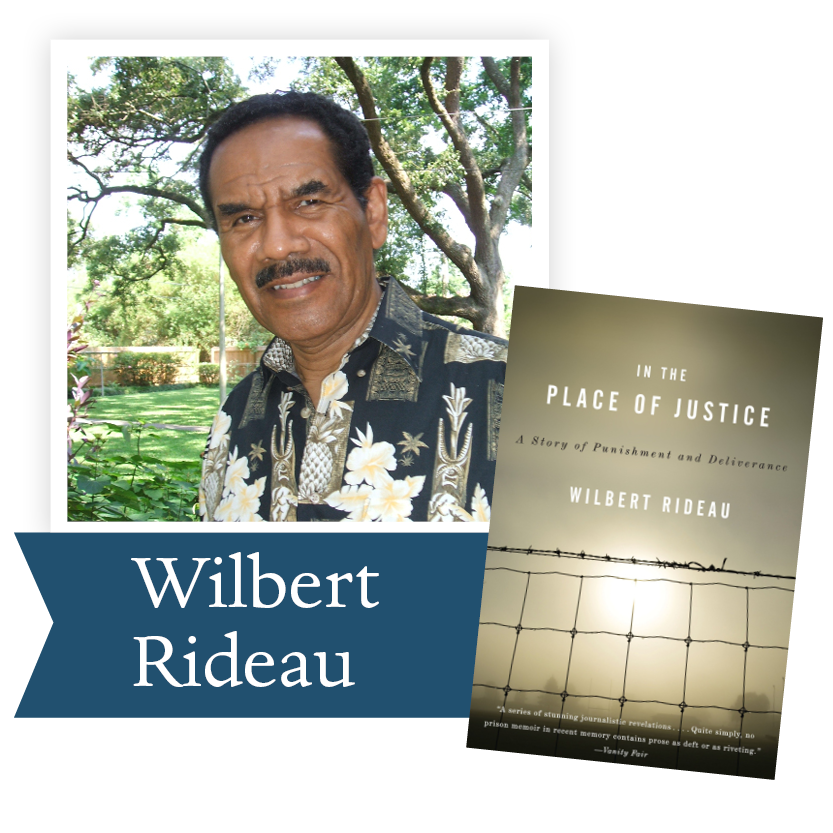In the Place of Justice: A Story of Punishment and Deliverance by Wilbert Rideau (Alfred A. Knopf): A death row inmate in Louisiana's Angola penitentiary, at the time the most violent in the nation, finds redemption as a prison journalist in this uplifting memoir.

 table div table+table+table+table+table+table+table+table+table+table+table+table+table+table+table+table+table+table+table+table+table+table+table+table+table+table+table+table+table+table+table+table div table{width:100%;padding:0}table div table+table+table+table+table+table+table+table+table+table+table+table+table+table+table+table+table+table+table+table+table+table+table+table+table+table+table+table+table+table+table+table div table img{width:96.23%;padding:0;float:none}table div table+table+table+table+table+table+table+table+table+table+table+table+table+table+table+table+table+table+table+table+table+table+table+table+table+table+table+table+table+table+table+table div table td{width:100%;padding:0 1.88% 18px}/* styles */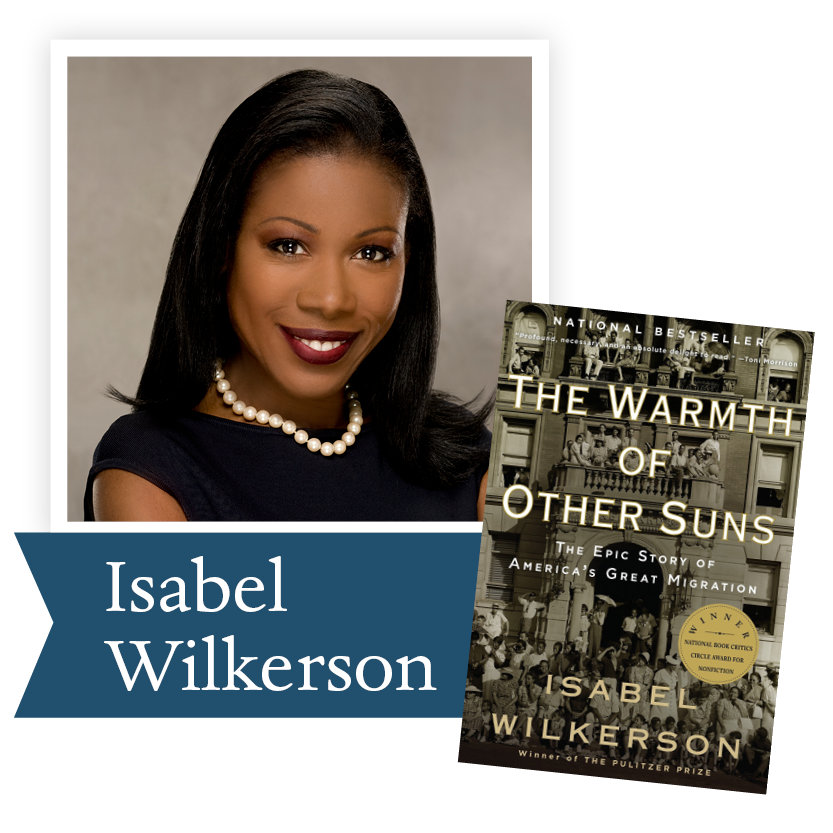The Warmth of Other Suns by Isabel Wilkerson (Random House): Wilkerson tells the stories of three black Americans who fled the South for an uncertain existence in the urban North and West in what became known as the Great Migration of the mid-20th century.

 table div table+table+table+table+table+table+table+table+table+table+table+table+table+table+table+table+table+table+table+table+table+table+table+table+table+table+table+table+table+table+table+table+table+table div table{width:100%;padding:0}table div table+table+table+table+table+table+table+table+table+table+table+table+table+table+table+table+table+table+table+table+table+table+table+table+table+table+table+table+table+table+table+table+table+table div table img{width:96.23%;padding:0;float:none}table div table+table+table+table+table+table+table+table+table+table+table+table+table+table+table+table+table+table+table+table+table+table+table+table+table+table+table+table+table+table+table+table+table+table div table td{width:100%;padding:0 1.88% 18px}/* styles */## 2011 Ambassador Richard C. Holbrooke Distinguished Achievement Award Winner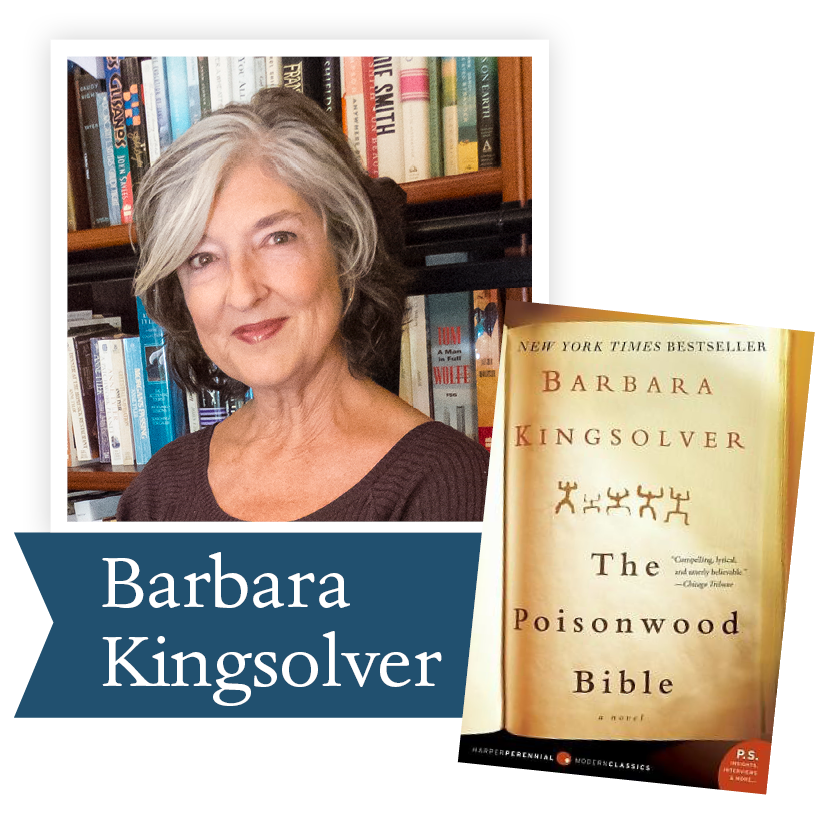Barbara Kingsolver charmed the audience at the gala by saying that she was delighted to celebrate peace in the heart of America, but she first charmed us with her novels. We are recommending three novels to you that will give you the sense of the scope of her work.

An early novel, The Bean Trees is a must read if you are new to Kingsolver’s work, or if you have read other works by her, but not this one. It is now a part of the canon in college literature classes across the nation.

We are also suggesting the work that many consider her masterpiece, The Poisonwood Bible. Set in the Belgian Congo in 1959, it follows with humor and insight into human failings a minister, his wife and his four daughters. This book has caught the imagination of the world.

One of her latest novels Unsheltered can speak to us during this difficult time. Published in 2018, this novel, “… is the compulsively readable story of two families, in two centuries, who live at the corner of Sixth and Plum in Vineland, New Jersey, navigating what seems to be the end of the world as they know it. With history as their tantalizing canvas, these characters paint a startlingly relevant portrait of life in precarious times when the foundations of the past have failed to prepare us for the future.”

 table div table+table+table+table+table+table+table+table+table+table+table+table+table+table+table+table+table+table+table+table+table+table+table+table+table+table+table+table+table+table+table+table+table+table+table+table+table div table{width:100%;padding:0}table div table+table+table+table+table+table+table+table+table+table+table+table+table+table+table+table+table+table+table+table+table+table+table+table+table+table+table+table+table+table+table+table+table+table+table+table+table div table img{width:96.23%;padding:0;float:none}table div table+table+table+table+table+table+table+table+table+table+table+table+table+table+table+table+table+table+table+table+table+table+table+table+table+table+table+table+table+table+table+table+table+table+table+table+table div table td{width:100%;padding:0 1.88% 18px}/* styles */table div table+table+table+table+table+table+table+table+table+table+table+table+table+table+table+table+table+table+table+table+table+table+table+table+table+table+table+table+table+table+table+table+table+table+table+table+table+table+table div table{width:100%;padding:0}table div table+table+table+table+table+table+table+table+table+table+table+table+table+table+table+table+table+table+table+table+table+table+table+table+table+table+table+table+table+table+table+table+table+table+table+table+table+table+table div table img{width:96.23%;padding:0;float:none}table div table+table+table+table+table+table+table+table+table+table+table+table+table+table+table+table+table+table+table+table+table+table+table+table+table+table+table+table+table+table+table+table+table+table+table+table+table+table+table div table td{width:100%;padding:0 1.88% 18px}/* styles */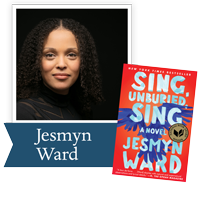Jesmyn Ward, a three-time finalist for the Dayton Literary Peace Prize, won the National Book Award for Sing, Unburied, Sing. Her work can educate us about the racial issues facing America today. Please join us in July to discuss this important book.

“The heart of Jesmyn Ward’s Sing, Unburied, Sing is story—the yearning for a narrative to help us understand ourselves, the pain of the gaps we’ll never fill, the truths that are failed by words and must be translated through ritual and song...Ward’s writing throbs with life, grief, and love, and this book is the kind that makes you ache to return to it.” —Buzzfeed

Ron Rollins, recently retired Dayton Daily News Ideas and Voices Editor, will moderate the discussion.

 table div table+table+table+table+table+table+table+table+table+table+table+table+table+table+table+table+table+table+table+table+table+table+table+table+table+table+table+table+table+table+table+table+table+table+table+table+table+table+table+table+table div table{width:100%;padding:0}table div table+table+table+table+table+table+table+table+table+table+table+table+table+table+table+table+table+table+table+table+table+table+table+table+table+table+table+table+table+table+table+table+table+table+table+table+table+table+table+table+table div table img{width:96.23%;padding:0;float:none}table div table+table+table+table+table+table+table+table+table+table+table+table+table+table+table+table+table+table+table+table+table+table+table+table+table+table+table+table+table+table+table+table+table+table+table+table+table+table+table+table+table div table td{width:100%;padding:0 1.88% 18px}/* styles */